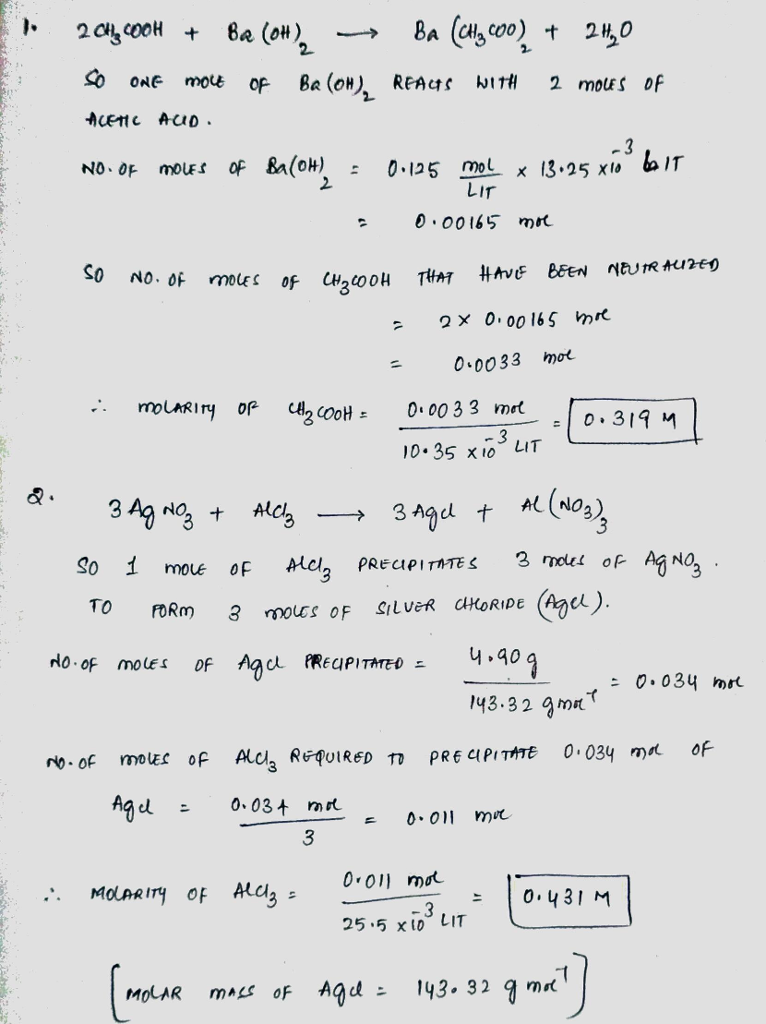# Homework Solution: In a neutralization reaction between acetic acid and barium hydroxide, 13.25 mL…

In a neutralization reaction between acetic acid and barium hydroxide, 13.25 mL of a 0.125M Ba(OH)_2 solution was required to neutralize 10.35 mL of an acetic acid solution. What is the molarity of the acetic acid solution? You will need to write and balance the equation for the neutralization reaction first. In a precipitation reaction between silver nitrate and aluminum chloride, 25.5 ml of an AlCl_3 solution was required to precipitate 4.90 g of silver chloride. What was the molarity of the AlCl_3 solution? You will need to write and balance the equation for the precipitation reaction first.